GetFEM  5.4.1
GetFEM reference documentation.# Introduction

This documentation has been generated from the GetFEM sources, this is not a tutorial.

# Terminology

This is just a short summary of the terms employed in getfem.

The mesh is composed of convexes. What we call convexes can be simple line segments, prisms, tetrahedrons, curved triangles, of even something which is not convex (in the geometrical sense). They all have an associated reference convex: for segments, this will be the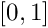segment, for triangles this will be the canonical triangle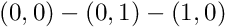etc... All convexes of the mesh are constructed from the reference convex through a geometric transformation. In simple cases (when the convexes are simplices for example), this transformation will be linear (hence it is easily inverted, which can be a great advantage). In order to define the geometric transformation, one defines geometrical nodes on the reference convex. The geometrical transformation maps these nodes to the mesh nodes.

On the mesh, one defines a set a basis functions: the FEM. A FEM should be associated with each convex. The basis functions are also attached to some geometrical points (which can be arbitrarily chosen). These points are similar to the mesh nodes, but they don't have to be the same (this only happens on very simple cases, such as a classical P1 fem on a triangular mesh). The set of all basis functions on the mesh forms the basis of a vector space, on which the PDE will be solved. These basis functions (and their associated geometrical point) are the degrees of freedom (dof). The FEM is said to be Lagrangian when each of its basis functions is equal to one at its attached geometrical point, and is null at the geometrical points of others basis functions. This is an important property as it is very easy to interpolate an arbitrary function on the finite elements space.

The finite elements methods involves evaluation of integrals of these basis functions (or product of basis functions etc...) on convexes (and faces of convexes). In simple cases (polynomial basis functions and linear geometrical transformation), one can evaluate analytically these integrals. In other cases, one has to approximate it, using quadrature formulas. Hence, at each convex is attached an integration method along with the FEM. If you have to use an approximate integration method, always choose carefully its order(i.e. highest degree of the polynomials who are exactly integrated with the method) : the degree of the FEM, of the polynomial degree of the geometrical transformation, and the nature of the elementary matrix have to be taken into account. If you are unsure about the appropriate degree, always prefer a high order integration method (which will slow down the assembly) to a low order one which will produce a useless linear-system.

The process of construction of a global linear system from integrals of basis functions on each convex is the assembly.

A mesh, with a set of FEM attached to its convexes is called a mesh_fem object in GetFEM.

A mesh, with a set of integration methods attached to its convexes is called a mesh_im object in GetFEM.

A mesh_fem can be used to approximate scalar fields (heat, pression, ..), or vector fields (displacement, electric field, ..). A mesh_im will be used to perform numerical integrations on these fields. Although GetFEM supports vector FEMs, intrinsic vector FEMs are essentially used in mixed methods (for instance Raviart-Thomas element). Most of the FEM currently available are scalar. Of course, these scalar FEMs can be used to approximate each component of a vector field. This is done by setting the Qdim of the mesh_fem to the dimension of the vector field (i.e. Qdim=1 for scalar field, Qdim=2 for 2D vector field etc...).

When solving a PDE, one often has to use more than one FEM. The most important one will be of course the one on which is defined the solution of the PDE. But most PDEs involve various coefficients, for example: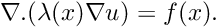Hence one has to define an FEM for the main unknown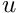, but also for the dataand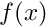if they are not constant. In order to interpolate easily these coefficients in their finite element space, one often choose a Lagrangian FEM.

The convexes, mesh nodes, and dof are all numbered. We sometimes refer to the number associated to a convex as its convex id or convex number. Mesh node numbers are also called point id or point number. Faces of convexes do not have a global numbering, but only a local number in each convex.

While the dof are always numbered consecutively, this is not always the case for point ids and convex ids, especially if you have removed points or convexes from the mesh. To ensure that they form a continuous sequence (starting from 0), you have to use the getfem::getfem_mesh::optimize_structure member function.

Most of the example programs of getfem now uses model bricks. Connecting these basic blocks one to each other, solvers for many PDE problems can be built.